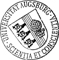## Front Motion in the One-Dimensional Stochastic Cahn-Hilliard Equation

• In this paper, we consider the one-dimensional Cahn-Hilliard equation perturbed by additive noise and study the dynamics of interfaces for the new stochastic model. The noise is smooth in space and is defined as a Fourier series with independent Brownian motions in time. Motivated by the work of Bates & Xun on slow manifolds for the integrated Cahn-Hilliard equation, our analysis reveals the significant difficulties and differences in comparison with the deterministic problem. New higher order terms, that we estimate, appear due to Itô calculus and stochastic integration dominating the exponentially slow deterministic dynamics of the interfaces. We derive a first order linear system of stochastic ordinary differential equations approximating the equations of front motion. Furthermore, we prove stochastic stability for the approximate slow manifold of solutions on a very long time scale and evaluate the noise effect.

• Dokument_1.pdfAuthor: Dimitra C. Antonopoulou, Dirk BlömkerORCiDGND, Georgia D. Karali urn:nbn:de:bvb:384-opus4-12076 https://opus.bibliothek.uni-augsburg.de/opus4/1498 Preprints des Instituts für Mathematik der Universität Augsburg (2011-05) Preprint English 2011 Universität Augsburg Department of Applied Mathematics, University of Crete, Institute of Applied and Computational Mathematics, FORTH 2011/03/21 Stochastische Differentialgleichunginterface motion; slow manifold; 1-D Stochastic Cahn-Hilliard; additive noise; dynamics Cahn-Hilliard-Gleichung; Stochastische nichtlineare Differentialgleichung; Stochastische partielle Differentialgleichung `Erschienen in SIAM Journal on Mathematical Analysis, 44, 5, S. 3242-3280, https://doi.org/10.1137/120861941` Mathematisch-Naturwissenschaftlich-Technische Fakultät Mathematisch-Naturwissenschaftlich-Technische Fakultät / Institut für Mathematik 5 Naturwissenschaften und Mathematik / 51 Mathematik / 510 MathematikDeutsches Urheberrecht mit Print on Demand